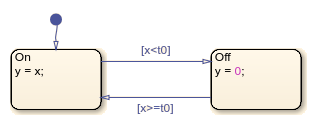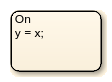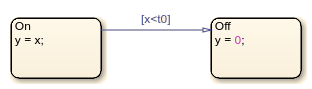# Create Charts by Using the Stateflow API

This example shows how to create a Stateflow® chart by using the Stateflow application programming interface (API). The Stateflow API is a tool to create or change Stateflow charts through MATLAB® commands. For more information, see Overview of the Stateflow API.

### Create a Stateflow Chart

This Stateflow chart presents the logic underlying a half-wave rectifier. The chart contains two states labeled `On` and `Off`. In the `On` state, the chart output signal `y` is equal to the input `x`. In the `Off` state, the output signal is set to zero. When the input signal crosses some threshold `t0`, the chart transitions between these states. The actions in each state update the value of `y` at each time step of the simulation.For more information on simulating this chart, see Construct and Run a Stateflow Chart.

1. Close all models.

`bdclose all`

2. Create a Simulink® model called `rectify` that contains an empty Stateflow Chart block.

`sfnew rectify`

### Access the `Chart` Object

To use the Stateflow API, you begin by accessing the `Simulink.Root` object, which is the parent of all objects in the Stateflow API. You use the `Simulink.Root` object to access the other API objects in your model.

1. Use the function `sfroot` to access the `Simulink.Root` object.

`rt = sfroot;`

2. Call the `find` function to access the `Stateflow.Chart` object that corresponds to the chart in your model.

`ch = find(rt,"-isa","Stateflow.Chart");`

3. To open the chart in the Stateflow Editor, call the `view` function.

`view(ch);`

4. To change the action language, modify the `ActionLanguage` property of the chart.

`ch.ActionLanguage = "C";`

To create a Stateflow API object as the child of a parent object, use the parent object as the input argument to a function that creates the child object. For more information, see Create and Delete Stateflow Objects.

1. Call the `Stateflow.State` function to add a state to the chart.

`s1 = Stateflow.State(ch);`

2. Adjust the position of the state by changing the `Position` property of the corresponding `State` object. Specify the new position as a four-element vector in which the first two values are the (x,y) coordinates of the upper-left corner of the state and the last two values are the width and height of the state.

`s1.Position = [30 30 90 60];`

3. Specify the name and label for the state by changing the `LabelString` property, as described in Specify Labels in States and Transitions Programmatically.

`s1.LabelString = "On"+newline+"y = x;";`4. Create a second state. Adjust its position and specify its name and label.

```s2 = Stateflow.State(ch); s2.Position = [230 30 90 60]; s2.LabelString = "Off"+newline+"y = 0;";```When you add a transition, you specify its source and destination by modifying its `Source` and `Destination` properties. For a default transition, you specify a destination but no source.

1. Call the `Stateflow.Transition` function to add a transition to the chart.

`t1 = Stateflow.Transition(ch);`

2. Set the transition source and destination.

```t1.Source = s1; t1.Destination = s2;```

3. Adjust the position of the transition by modifying its `SourceOClock` property.

`t1.SourceOClock = 2.1;`

4. Specify the transition label and its position by changing the `LabelString` and `LabelPosition` properties.

```t1.LabelString = "[x<t0]"; t1.LabelPosition= [159 23 31 16];```5. Create a second transition. Specify its source, destination, and label.

```t2 = Stateflow.Transition(ch); t2.Source = s2; t2.Destination = s1; t2.SourceOClock = 8.1; t2.LabelString = "[x>=t0]"; t2.LabelPosition= [155 81 38 16];```6. Add a default transition to the state `On`. To make a vertical transition, modify the values of the `SourceEndpoint` and `Midpoint` properties. For more information, see Add a Default Transition.

```t0 = Stateflow.Transition(ch); t0.Destination = s1; t0.DestinationOClock = 0; t0.SourceEndpoint = t0.DestinationEndpoint-[0 30]; t0.Midpoint = t0.DestinationEndpoint-[0 15];```Before you can simulate your chart, you must define each data symbol that you use in the chart and specify its scope and type.

1. Call the `Stateflow.Data` function to add a data object that represents the input to the chart.

`x = Stateflow.Data(ch);`

2. Specify the name of the data object as `x` and its scope as `Input`.

```x.Name = "x"; x.Scope = "Input";```

3. To specify that the input `x` has type `double`, set its `Props.Type.Method` property to `Built-in`. The default built-in data type is `double`.

```x.Props.Type.Method = "Built-in"; x.DataType```
```ans = 'double' ```

4. Add a data object that represents the output for the chart. Specify its name as `y` and its scope as `Output`.

```y = Stateflow.Data(ch); y.Name = "y"; y.Scope = "Output";```

5. To specify that the output y has type `single`, set its `Props.Type.Method` property to `Built-in` and its `DataType` property to `single`.

```y.Props.Type.Method = "Built-in"; y.DataType = "single"; y.DataType```
```ans = 'single' ```

6. Add a data object that represents the transition threshold in the chart. Specify its name as `t0` and its scope as `Constant`. Set its initial value to `0`.

```t0 = Stateflow.Data(ch); t0.Name = "t0"; t0.Scope = "Constant"; t0.Props.InitialValue = "0";```

7. To specify that the threshold `t0` has a fixed-point data type, set its `Props.Type.Method` property to `Fixed-point`. Then specify the values of the `Props.Type` properties that apply to fixed-point data.

```t0.Props.Type.Method = "Fixed point"; t0.Props.Type.Signed = true; t0.Props.Type.WordLength = "5"; t0.Props.Type.Fixpt.ScalingMode = "Binary point"; t0.Props.Type.Fixpt.FractionLength = "2"; t0.DataType```
```ans = 'fixdt(1,5,2)' ```

### Save and Simulate Your Chart

To save the model that contains your completed chart, call the `sfsave` function.

`sfsave`

To simulate the chart, connect it to other blocks in the Simulink model through input and output ports.